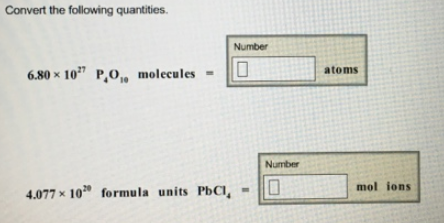# Problem: Convert the following quantities.6.80 x 1027 P4O10 molecules = 4.077 × 1020 formula units PbCl4 =

###### FREE Expert Solution###### Problem Details

Convert the following quantities.

6.80 x 1027 P4O10 molecules =

4.077 × 1020 formula units PbCl4 =